Determining Temperature from Density and Pressure

 |   Tiled Menu   |   Tables of Content   |  Banner Video   |  Tohline Home Page   |

As has been derived elsewhere, the normalized total pressure can be written as,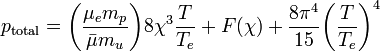$~p_\mathrm{total} = \biggl(\frac{\mu_e m_p}{\bar{\mu} m_u} \biggr) 8 \chi^3 \frac{T}{T_e} + F(\chi) + \frac{8\pi^4}{15} \biggl( \frac{T}{T_e} \biggr)^4$

To solve this algebraic equation for the normalized temperature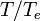$~T/T_e$, given values of the normalized total pressure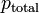$~p_\mathrm{total}$ and the normalized density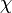$~\chi$, we first realize that the equation can be written in the form,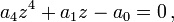$~ a_4z^4 + a_1 z - a_0 = 0 \, ,$

where,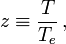$~z \equiv \frac{T}{T_e} \, ,$

and the coefficients,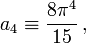$~ a_4 \equiv \frac{8\pi^4}{15} \, ,$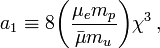$~ a_1 \equiv 8\biggl(\frac{\mu_e m_p}{\bar{\mu} m_u} \biggr) \chi^3 \, ,$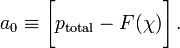$~ a_0 \equiv \biggl[p_\mathrm{total} - F(\chi) \biggr] \, .$

Mathematical Manipulation

Quartic Equation Solution

Following the Summary of Ferrari's method that is presented in Wikipedia's discussion of the Quartic Function to identify the roots of an arbitrary quartic equation, we can set the coefficients of the cubic and quadratic terms both to zero and deduce that the only root that will give physically relevant temperatures — for example, real and non-negative — is,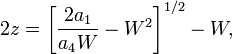$2z = \biggl[\frac{2a_1}{a_4 W}-W^2\biggr]^{1/2} - W ,$

where,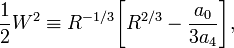$\frac{1}{2}W^2 \equiv R^{-1/3}\biggl[R^{2/3} - \frac{a_0}{3a_4} \biggr] ,$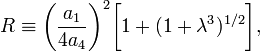$R \equiv \biggl( \frac{a_1}{4a_4} \biggr)^2 \biggl[ 1 + (1 + \lambda^3)^{1/2} \biggr] ,$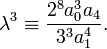$\lambda^3 \equiv \frac{2^8 a_0^3 a_4}{3^3 a_1^4} .$

Defining,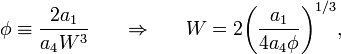$\phi \equiv \frac{2a_1}{a_4 W^3} ~~~~~\Rightarrow ~~~~~ W = 2\biggl(\frac{a_1}{4a_4 \phi} \biggr)^{1/3} ,$

and realizing that, from one of the above expressions,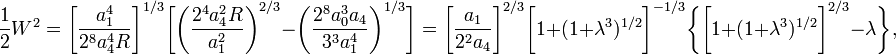$\frac{1}{2}W^2 = \biggl[ \frac{a_1^4}{2^8 a_4^4 R}\biggr]^{1/3}\biggl[\biggl( \frac{2^4 a_4^2 R}{a_1^2}\biggr)^{2/3} - \biggl( \frac{2^8 a_0^3 a_4}{3^3 a_1^4} \biggr)^{1/3} \biggr] = \biggl[ \frac{a_1}{2^2 a_4}\biggr]^{2/3} \biggl[ 1 + (1 + \lambda^3)^{1/2} \biggr]^{-1/3}\biggl\{ \biggl[ 1 + (1 + \lambda^3)^{1/2} \biggr]^{2/3} - \lambda \biggr\} ,$

we can rewrite the desired root of our quartic equation in the form,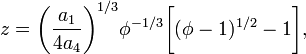$z = \biggl(\frac{a_1}{4a_4}\biggr)^{1/3} \phi^{-1/3}\biggl[ (\phi - 1)^{1/2} - 1 \biggr] ,$

with,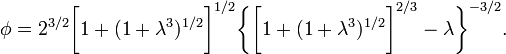$\phi = 2^{3/2} \biggl[ 1 + (1 + \lambda^3)^{1/2} \biggr]^{1/2} \biggl\{ \biggl[ 1 + (1 + \lambda^3)^{1/2} \biggr]^{2/3} - \lambda \biggr\}^{-3/2} .$

Key Dimensionless Parameters

From the above solution, there appear to be two key dimensionless parameters that can be formed from a strategic combination of the coefficients of the original quartic equation. They are,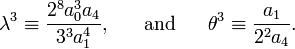$~ \lambda^3 \equiv \frac{2^8 a_0^3 a_4}{3^3 a_1^4} , ~~~~~\mathrm{and}~~~~~ \theta^3 \equiv \frac{a_1}{2^2 a_4} .$

[Note, as well, that the product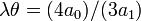$~\lambda\theta = (4a_0)/(3a_1)$.] The desired solution of our quartic equation is a product of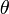$~\theta$ and an expression that is only a function of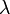$~\lambda$. Specifically, we can write,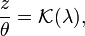$~ \frac{z}{\theta} = \mathcal{K}(\lambda) ,$

where, in terms of the above-defined function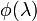$~\phi(\lambda)$,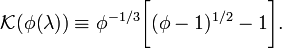$~ \mathcal{K}(\phi(\lambda)) \equiv \phi^{-1/3}\biggl[ (\phi - 1)^{1/2} - 1 \biggr] .$

Limiting Regimes

We can immediately see that this solution makes sense in the present context. In order for the temperature — that is,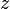$~z$ — to be real and nonnegative, the function$~\phi(\lambda)$ must be greater than or equal to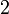$~2$. This limiting value occurs when the dimensionless parameter,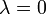$~\lambda = 0$. The constraint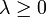$~\lambda \ge 0$ is satisfied as long as the three coefficients of the quartic equation are real and nonnegative, which is certainly true for our specific problem.

Looking at the limiting functional behavior of our solution, we see that when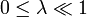$~0 \le \lambda \ll 1$,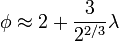$~ \phi \approx 2 + \frac{3}{2^{2/3}}\lambda$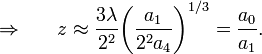$~ \Rightarrow ~~~~~ z \approx \frac{3\lambda}{2^2} \biggl( \frac{a_1}{2^2a_4} \biggr)^{1/3} = \frac{a_0}{a_1} .$

We see, as well, that when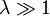$~\lambda \gg 1$,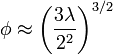$~ \phi \approx \biggl(\frac{3\lambda}{2^2} \biggr)^{3/2}$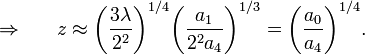$~ \Rightarrow ~~~~~ z \approx \biggl(\frac{3\lambda}{2^2} \biggr)^{1/4} \biggl( \frac{a_1}{2^2a_4} \biggr)^{1/3} = \biggl( \frac{a_0}{a_4} \biggr)^{1/4} .$

Physical Implications

Clearly, a key dimensionless physical parameter for this problem is,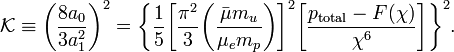$~ \mathcal{K} \equiv \biggl( \frac{8a_0}{3a_1^2} \biggr)^2 = \biggl\{ \frac{1}{5} \biggl[ \frac{\pi^2}{3} \biggl(\frac{\bar{\mu} m_u}{\mu_e m_p} \biggr) \biggr]^2 \biggl[ \frac{p_\mathrm{total} - F(\chi)}{\chi^6} \biggr] \biggr\}^2 .$

And, because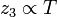$~z_3 \propto T$ and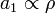$~a_1 \propto \rho$, the above solution tells us that the product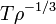$~T \rho^{-1/3}$ can be expressed as a function of this single parameter,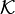$~\mathcal{K}$.